# tixy.land

Yesterday I discovered this tweet from Martin Kleppe, which introduces the tixy.land website:

The idea is quite fun: basically, you can write a math expression that depends only on 4 variables (t,i,x,y) (hence the name of the web page), and the expression is rendered as animated dots located on a 16x16 grid, by applying simple rendering rules. Like this (example taken from the website):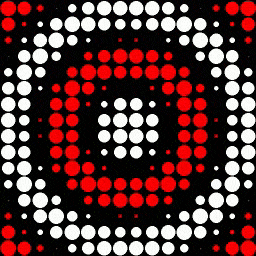The goal is then to provide a math expression that renders the “best” possible animation. And as the expression can be encoded directly in the URL, you can share it with others.
That’s pretty cool!

A lot of people actually replied to the initial tweet with examples of expressions that has become more and more complex in the evening. The expression given by the user is actually written in Javascript. I know almost nothing about Javascript, but I guess complex mini-programs can be written as math expressions. The site tells us that the expressions are limited to 32 chars, but that’s true only when you enter them on the text input form provided on the website. You can cheat a bit if you encode the expression directly in the URL.

The idea pleased me a lot, and I decided to give a try. To make my tests easier, I’ve recoded a G’MIC command that renders the same kind of animation than the tixy.land website. This command is usable from the CLI interface `gmic` of G’MIC, and should be available after a stdlib update, if you use G’MIC 2.9.0+ (so `\$ gmic up` and you’re ready).
The command allows you to save a `.avi` file corresponding to the animation, and is able to export the specified expression directly as a tixy.land URL.

That’s useful to test math expressions locally, without having to test directly on the tixy.land website. The grammar is not exactly the same, as here, I use the G’MIC math parser to evaluate the expressions, but it’s really not so different than what I’ve seen in Javascript so far.

# My first attempt:

I wanted to do something quite complex, so I didn’t try to respect the 32 chars limit.

What I did is take the `heart` binary shape (G’MIC command `shape_heart`), resized it to 16x16 and applied the DCT.
Then I filtered the DCT coefficients to keep only those with a high magnitude. As a result, I got an analytic expression, as a sum of weighted cosines, which renders an approximation of the heart binary shape. I finally added the (allowed) time variable `t` in some of the cosines (those with the lowest coefficients), to generate an animation of a beating heart.

Here is the expression I ended up with:

``````expr = cos(0.4*x-0.2)+0.3*cos(0.8*x-0.4)-cos(0.2*y-0.1)-0.7*cos(0.4*x-0.2)*cos(0.2*y-0.1)+0.7*cos(0.8*x-0.4)*cos(0.2*y-0.1)+1.7*cos(0.4*y-0.2)-0.3*cos(0.4*x-0.2+3*t)*cos(0.4*y-0.2-t)+0.7*cos(0.6*y-0.3)+0.6*cos(0.4*x-0.2)*cos(0.6*y-0.3)+0.3*cos(0.8*x-0.4+t)*cos(0.6*y-0.3)+0.5*cos(0.8*y-0.4)+0.3*cos(1.2*x-0.6)*cos(0.8*y-0.4)+0.4*cos(y-0.5)-0.5*cos(0.8*x-0.4)*cos(y-0.5)-0.3*cos(1.6*x-0.8)*cos(1.2*y-0.6)-0.3*cos(0.8*x-0.4)*cos(1.6*y-0.8)
``````

And here is the resulting animation: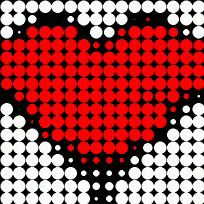What is nice is that you can see the same animation by visiting the following URL, redirecting to the tixy.land website:

https://tixy.land?code=cos%280.4x-0.2%29%2B0.3cos%280.8x-0.4%29-cos%280.2y-0.1%29-0.7cos%280.4x-0.2%29cos%280.2y-0.1%29%2B0.7cos%280.8x-0.4%29cos%280.2y-0.1%29%2B1.7cos%280.4y-0.2%29-0.3cos%280.4x-0.2%2B3t%29cos%280.4y-0.2-t%29%2B0.7cos%280.6y-0.3%29%2B0.6cos%280.4x-0.2%29cos%280.6y-0.3%29%2B0.3cos%280.8x-0.4%2Bt%29cos%280.6y-0.3%29%2B0.5cos%280.8y-0.4%29%2B0.3cos%281.2x-0.6%29cos%280.8y-0.4%29%2B0.4cos%28y-0.5%29-0.5cos%280.8x-0.4%29cos%28y-0.5%29-0.3cos%281.6x-0.8%29cos%281.2y-0.6%29-0.3cos%280.8x-0.4%29cos%281.6*y-0.8%29

That’s all for now, but I’m sure I will give another try in the future.

And you, what would you be able to achieve ?8 Likes
1. Many programmers are ill by design. Sorry to say but it’s the naked truth.
2. Often they make fascinating software, like this tixiland thing. Eager to explore more.

Thanks David for sharing this half-crazy thing.A bit more of thisI thought of a “simple” way to add colors to those `pixy.land` animations, without having to write mathematical expressions that would generate 4D vectors, namely `[r,R,G,B]` , where `r` would be the radius of the disk, and `[R,G,B]` its color, defined at each point.
On the contrary, we want to be able to keep mathematical expressions simple, and be amazed to see that it generates complicated animationsAlso, the constraint I imposed on myself was to get the same animations as on `tixy.land`, with the same previous formulas.

So I simply considered that mathematical expressions should return complex numbers `C` rather than real numbers, with the following rules:

• the module `cabs(C)` of the complex number `C` determines the radius of the disk at each point `(x,y)`.
• The argument `carg(C)` of the complex number `C` determines its color, in the form of a hue (the argument can be seen as an angle, so the HSV space is particularly adapted here).

I’ve also added a new variable `z`, shortcut for `z = x + j*y`, where `j` is the imaginary number `[0,1]` (`i` was already used in `tixy.land` expressions).

What is cool is that the original colored scheme is preserved : if the expression returns a real number (i.e. a complex `C = [ r,0 ]`), then `cabs(C)` = `abs(r)` is indeed the radius of the disk, and `carg(C) = r>=0?0:pi` so two different colors are still mapped regarding to the sign of `r`.
So, we get an equivalence with the previous (real-valued) formulas. This is nice.

Now, let me show you some simple examples below. As you see, the formulas remain simple, and we got full color rendering. Yipeee!!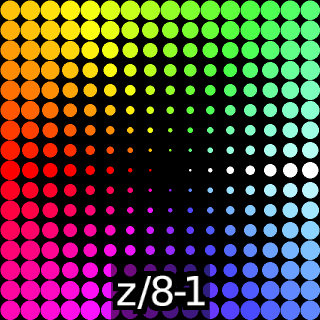with the time variable `t` introduced: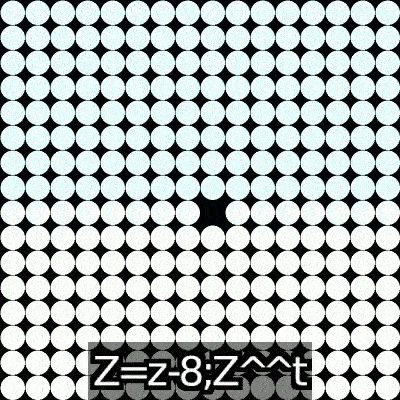And another one:

Nice, isn’t it ?4 Likes

This is AWESOME!

Fascinating !
Creating a short word is beyond the scope of this 16x16 thing, I suppose…?

Like this ?

``````\$ gmic tixy "[[62551,34516,38228,62551,0,0][(y+10*t)%6]&(32768>>x),0.5*cos(t)]"
``````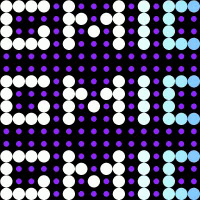2 Likes

Amazing ! I’ll dig into this later, thanks for pointing me in this direction…

This reminds me of Shadertoy, but minimalistic. Perhaps the information on this website page from Inigo Quilez (one of the creators of Shadertoy) is interesting for new ideas on a small scale too? Or does this have no similarities with the thoughts behind tixy.land?